### IMO Shortlist 1987 problem 7

Kvaliteta:
Avg: 0,0
Težina:
Avg: 0,0
Given five real numbers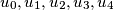$u_0, u_1, u_2, u_3, u_4$, prove that it is always possible to find five real numbers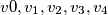$v0, v_1, v_2, v_3, v_4$ that satisfy the following conditions: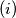$(i)$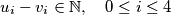$u_i-v_i \in \mathbb N, \quad 0 \leq i \leq 4$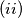$(ii)$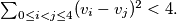$\sum_{0 \leq i

Proposed by Netherlands.
Izvor: Međunarodna matematička olimpijada, shortlist 1987